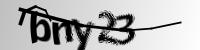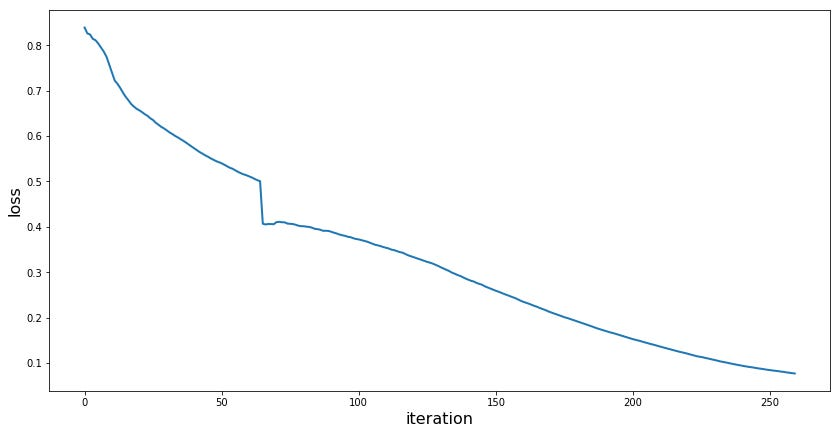# Solving Captchas with DeepLearning — Part 1: Multi-label Classification

Source: Deep Learning on Medium

Having completed lessons 1–4 from the fast.ai “Practical DeepLearning for Coders” I decided to try a project on my own. The lessons were heavy on computer vision, so I decided to find a project in that direction.

The notebook can be found here.

After some thinking I remembered something I haven’t seen for a while: Captchas. Those squiggly letters and numbers, distorted and with added obfuscation so that no human could possibly identify what it’s supposed to be. With the impressive results that are achieved on a daily basis by CNN’s for computer vision I decided to give it a try.

This is the first in a series of posts. I will introduce the dataset and show a proof of concept that CNN’s are suited to solve this kind of task. The following posts (1 or 2 planned) will show how to get a real working solution for automated captcha recognition.

### Dataset

Fortunately, there was this dataset on kaggle that provided 1070 labeled captchas. They look like this:I’m sure that I wouldn’t get a 100% on these…

Looking through the dataset, I found it to have the following properties:

• Each captcha consists of exactly 5 characters
• A character is either a letter (a-z) or a number (1–9)
• A character might appear multiple times in a single captcha

The data is given as a folder containing all the captcha images. The label for each image is given by its filename. ‘bny23.png’ for the example above. This allows for easy extraction of the labels when preparing the dataset:

`def label_from_filename(path): label = [char for char in path.name[:-4]] return label`

### Multi-Label Classification

In multi-label classification we have a list of labels. When given an input image, we’re trying to predict which of the labels the input image has. An image can have multiple labels.

In the case of captcha solving, the list of labels would look like this:

• Has an ‘a’ in it
• Has a ‘b’ in it
• Has a ‘1’ in it
• ….

Multi-Label classification allows us to tell which characters are present in the captcha. The obvious shortcommings of this approach are:

• We don’t know at which position each character is
• We don’t know how many instances of each character there is.

Still this is a useful first step to get an idea if a CNN is able to handle the task at all.

So how does the idea of multi-label classification translate into the architecture of the CNN?

As usual, we start of with some convolutional layers. Beginning with the original image, a number of convolutional filters is applied. The output is a set of feature maps that detect simple features, like straight lines. In each of the following layers, another set of convolutional filters is applied to the feature maps produced by the previous layer. The complexity of the detected features increases as more and more layers are stacked upon each other.

At the end we end up with scalar features. The elements of the last layer are no longer maps (2 dimensional) but scalars (“normal numbers”, 1 dimensional). These numbers can be thought of as automatically extracted features that describe some properties of the input image.

Those extracted features are then used as input to a fully connected neural network. The FCNN functions as a classificator acting on the features extracted by the convolutional layers.

The FCNN has one output for each label. Each output can be thought of as a probability that the input image has this label. In the example above, the output is interpreted as “The captcha contains B and 2”.

Fortunately the fast.ai library makes it very easy to create such a structure. First, we load the data via the datablock api:

`data = (ImageList.from_folder(path) .split_by_rand_pct(0.2) .label_from_func(label_from_filename) .transform(get_transforms(do_flip=False)) .databunch() .normalize() )`

This results in a dataset like this:

Notice that the captcha in the top left has two ‘d’ in it, but the label only has one. This is in line with the idea of “the captcha has (at least) a d in it”.

For the actual training we’ll use transfer learning. The main idea is to use a feature extractor that was trained on a large dataset. On top of that, a custom FCNN is added for classification.

In the first step, we only train the custom classification layers at the end. Since their weights are completely random in the beginning, training them together with the pre-trained feature extractor layers would just mess them up.

`learn = cnn_learner(data, models.resnet18, model_dir='/tmp')`
`lr = 5e-2learn.fit_one_cycle(5, lr)`

Once the custom classification layers give good results, we “unfreeze” the network. That means, from now on every layer is trained. This gives the possibility to adjust the features that are detected.

`learn.unfreeze()learn.fit_one_cycle(15, slice(1e-3, lr/5))`

The final accuracy on the validation set is above 98%.

Plotting the loss of the model over the iterations clearly shows the unfreezing point. It’s a sudden decline in loss:Clearly visible jump at around iteration 60

For each input image from the validation set, a prediction was made. Comparing the prediction against the actual label allows us to calculate the loss. The higher the loss, the “more wrong” the prediction was. With this, we can view the inputs with the largest loss, the captchas that were the “most wrong”: